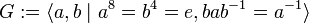# Semidirect product of Z8 and Z4 of dihedral type

View a complete list of particular groups (this is a very huge list!)[SHOW MORE]

## Definition

This group is defined by the following presentation:$\! G := \langle a,b \mid a^8 = b^4 = e, bab^{-1} = a^{-1} \rangle$

## Arithmetic functions

Want to compare and contrast arithmetic function values with other groups of the same order? Check out groups of order 32#Arithmetic functions

## GAP implementation

### Group ID

This finite group has order 32 and has ID 14 among the groups of order 32 in GAP's SmallGroup library. For context, there are 51 groups of order 32. It can thus be defined using GAP's SmallGroup function as:

SmallGroup(32,14)

For instance, we can use the following assignment in GAP to create the group and name it$G$:

gap> G := SmallGroup(32,14);

Conversely, to check whether a given group$G$ is in fact the group we want, we can use GAP's IdGroup function:

IdGroup(G) = [32,14]

or just do:

IdGroup(G)

to have GAP output the group ID, that we can then compare to what we want.

### Description by presentation

gap> F := FreeGroup(2);
<free group on the generators [ f1, f2 ]>
gap> G := F/[F.1^8,F.2^4,F.2*F.1*F.2^(-1)*F.1];
<fp group on the generators [ f1, f2 ]>
gap> IdGroup(G);
[ 32, 14 ]